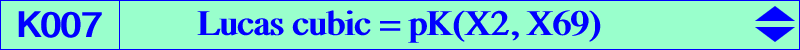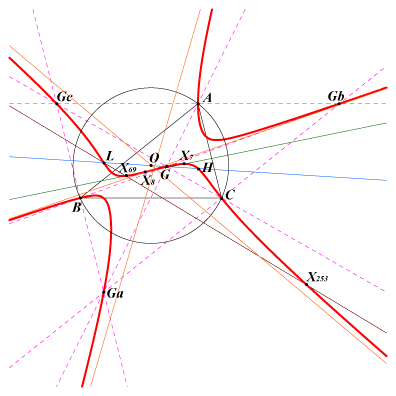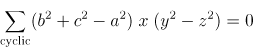X(2), X(4), X(7), X(8), X(20), X(69), X(189), X(253), X(329), X(1032), X(1034), X(5932), X(14361), X(14362), X(14365), X(34162), X(39158), X(39159), X(39160), X(39161), X(41080), X(42427), X(42428) X(55830) → X(55837) vertices of the 2nd Conway triangle, see ETC X(9776) vertices of antimedial triangle vertices of the Lucas triangle, see below foci of the Steiner circum-ellipse (see below) points at infinity of the Thomson cubicCPCC or H-cevian points, see Table 11, and their isotomic conjugates seven central cyclocevian points, see Table 24. ***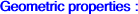The Lucas cubic is the isotomic pK with pivot X(69), isotomic conjugate of H. It is the Thomson cubic of the antimedial triangle. See K878 for a generalization and other related cubics. Its isogonal transform is K172. It is a member of the classes CL023, CL024 of cubics. See also Table 15. It is anharmonically equivalent to the Thomson cubic. See Table 21. Locus properties : Locus of point P whose cevian triangle is a pedal triangle. Locus of point P whose cevian triangle is orthologic to ABC. The locus of the centers of orthology is the Darboux cubic. See a generalization at CL023. Locus of point P whose cevian triangle is orthologic to the medial triangle. The locus of the centers of orthology is the Darboux cubic and its complement. See a generalization at Table 7. Locus of point P whose cevian triangle is orthologic to the antimedial triangle. The locus of the centers of orthology is the Darboux cubic. PaPbPc is the cevian triangle of (finite) point P. For any real k, the homothety h(P,k) maps PaPbPc to QaQbQc. The triangles QaQbQc and ABC are orthologic (for all k) if and onky if P lies on the Lucas cubic. (Fred Lang, Hyacinthos #5849). Locus of point P such that the cevian triangles of P and its isotomic conjugate P' are orthologic. The locus of the centers of orthology is K401. Let P be the perspector of an inscribed conic (C) with center Q. The normals at the contacts of (C) with the sidelines of ABC concur if and only if P lies on the Lucas cubic and then, Q lies on the Thomson cubic K002. In this case, the circum-conic (C') passing through P and Q is a rectangular hyperbola. HaHbHc is the orthic triangle and MaMbMc the cevian triangle of M. Da is the line passing through Ma and the midpoint of AHa, Db and Dc similarly. These lines are concurrent (at Q) if and only if M lies on the Lucas cubic. The locus of Q is the Thomson cubic. (Philippe Deléham, 17 nov. 2003) More generally, if HaHbHc is replaced by the cevian triangle of point P, the locus of M is pK(X2,tP) where tP is the isotomic conjugate of P, and the locus of Q is pK(ctP, X2) where ctP is the complement of tP. For example, with P = X(67), we find K008 and K043 respectively and with P = X(69), we find K170 and K168 respectively. The cevian lines of P meet the Steiner ellipse again at A', B', C'. The pedal triangle of P and A'B'C' are perspective if and only if P lies on K007. See also CL024. Locus of point P such that the trilinear polar of P is perpendicular to the line PL where L = X(20) = de Longchamps point. See CL040 for a generalization. Locus of the {X}-cevian points where X is a center on the Thomson cubic. See Table 28 : cevian and anticevian points. Let (C) be the inconic with perspector P and H(A), H(B), H(C) the Apollonius hyperbolas of A, B, C with respect to (C). The centers of these hyperbolas form a triangle perspective to ABC if and only if P lies on the Lucas cubic (Angel Montesdeoca, 2010-03-03). If A'B'C' is the cevian triangle of X then K007 is the locus of X for which BA'^2 + CB'^2 + AC'^2 = A'C^2 + B'A^2 + C'B^2. Compare with K034 and K200. Let A' B' C' be the cevian triangle of a point P. The circumcircles (Oa), (Ob), (Oc) of AB'C', BC'A', CA'B' are concurrent at M. Denote by  O* the circumcenter  of OaObOc. K007 is the locus of point P such that M, O*, O are collinear (or M is the reflection of O in O*). When P varies on the Lucas cubic, the locus of M is the Darboux cubic K004 (Angel Montesdeoca, private message 2013-08-06). See the related cubic K279. Let PaPbPc be the cevian triangle of a point P and MaMbMc the medial triangle of PaPbPc. ABC and MaMbMc are orthologic when P varies on the Lucas cubic. The loci of the centers of orthology are the Darboux cubic K004 and the Burek-Hutson central cubic K645. (Angel Montesdeoca, private message 2016-09-02). Other related properties to be found in the page K645. Let A'B'C' be the cevian triangle of a point U. Denote by f the affine application that transforms ABC into A'B'C', whose finite fixed point F is the crosspoint of the centroid G and U. When a point M traverses an arbitrary line ℓ, the line Mf(M) envelopes a parabola P(ℓ). K007 is the locus of point U such that the directrix of P(ℓ) passes through F (for all ℓ). (Angel Montesdeoca, private message 2019-03-23). Let PaPbPc be the cevian triangle of a point P. Denote by Ab the intersection of the line AP with the perpendicular at Pb to AC and by Ac the intersection of the line AP with the perpendicular at Pc to AB. Bc, Ba and Ca, Cb are defined cyclically. The lines AbBa, BcCb, CaAc concur (at Q) if and only if P lies on K007 (Angel Montesdeoca, 2019-07-24, see here in Spanish). The locus of Q is a circum-sextic passing through X(2), X(4), X(141), X(226), X(394), X(6736), X(13155), X(13156), X(13157), the vertices of the cevian triangle of X(69), the infinite points of the medians of ABC, the infinite points of pK(X2, X317) and pK(X6, X6515). X(4) is a quadruple point. Here is a list of pairs {P, Q} : {2, 141}, {4, 4}, {7, 226}, {8, 6736}, {20, 13155}, {69, 394}, {189, 13156}, {253, 13157}. Let PaPbPc be the cevian triangle of a point P and MaMbMc the medial triangle of ABC. Denote by Oa, Ob, Oc the circumcenters of APbPc, BPcPa, CPaPb. The triangles OaObOc and MaMbMc have equal areas when P lies on K007 in which case ABC and OaObOc are homothetic at a point on K004. Otherwise, the area of OaObOc is greater than that of MaMbMc (Kadir Altintas). Let P be a point with cevian triangle PaPbPc. Denote by Ba and Ca the reflections of B and C in Pa respectively. Define Cb, Ab and Ac, Bc cyclically. (Ca) is the circumcircle of triangle ABcCb and (Cb), (Cc) are defined likewise. These three circles are congruent if and only if P lies on K007 (Kadir Altintas, 2021-01-16). Furthermore, the radical center Q of (Ca), (Cb), (Cc) lies on the line PX(20) and on K004. Q is the barycentric product cgP x agctP. If Oa, Ob, Oc are the centers of these circles, the triangle OaObOc is the image of ABC under the translation that maps O to Q. Let PaPbPc be the cevian triangle of a point P. Let La be the radical axis of the circles (Pb, BPc) and (Pc, CPb), and define Lb and Lc similarly. The lines La, Lb, Lc concur if and only if P lies on K007 (Angel Montesdeoca, 2021-04-21).Transformations on the Lucas cubic and associated group law The Lucas cubic is invariant under isotomic conjugation and under several other transformations. Its is indeed the locus of point P such that : X(69), P and its isotomic conjugate tP are collinear (since X(69) is the pivot), X(4), P and X69/P = X(69)-Ceva conjugate of P are collinear (since X(4) is the isopivot), X(14361), P and X4©P = X(4)-crossconjugate of P are collinear (since X(14361) is the X(69)-Ceva conjugate of X(4)), X(1032), the X(69)-Ceva conjugates of P and tP are collinear, X(20), P and cccP = cyclocevian conjugate of P are collinear, X(2), P and agcP = anticomplement of the isogonal of the complement of P are collinear (derives from the definition of the Thomson cubic K002). This point agcP is also the X(1)-anticomplementary conjugate of P. As in the pages K002 and K004, a group law is defined on K007 with neutral element X(69). Each center P on K007 is associated with an integer n. Three points are collinear if and only if the sum of the corresponding integers is 4. Q is the tangential of P.n -10 -9 -8 -7 -6 -5 -4 -3 -2 -1 0 P X(55832) X(55837) X(55830) X(55831) X(14362) X(34162) X(14361) X(5932) X(20) X(329) X(69) Q X(55833) X(14365) X(1032) X(253) X(4)0 1 2 3 4 5 6 7 8 9 10 11 X(69) X(8) X(2) X(7) X(4) X(189) X(253) X(1034) X(1032) X(41080) X(14365) X(55836) X(4) X(2) X(69) X(20) X(14361) X(14362) X(55830) X(55832)If P and P' are two points with integers n and n' then : • P and P' are X(69)-Ceva conjugates hence collinear with X(4) if and only if n + n' = 0, • P and P' are agc conjugates hence collinear with X(2) if and only if n + n' = 2, • P and P' are isotomic conjugates hence collinear with X(69) if and only if n + n' = 4, • P and P' are cyclocevian conjugates hence collinear with X(20) if and only if n + n' = 6, • P and P' are X(4)-cross conjugates hence collinear with X(14361) if and only if n + n' = 8. The tangential Q of P corresponds to 4 - 2n hence the isotomic conjugate of Q corresponds to 2n. For example, the point P(-5) = X(34162) = X69/X189 = agcX1034 = tP(9). P(-5) and P(9) are the anticomplements of X(3342) and X(3351). Similarly, P(-8), P(-7), P(-10) and P(12) are the anticomplements of X(3349), X(3352), X(3356) and X(14481) respectively.The Lucas cubic and the foci of the Steiner ellipse The Lucas cubic is the most familiar example of isocubic which contains the (four) foci of the Steiner (circum) ellipse. See also K347 and K348. Indeed, these foci are the anticomplements of those of the inscribed Steiner ellipse which lie on the Thomson cubic since they are two isogonal conjugates collinear with the pivot G. Let then be F1, F2 the real foci and F1', F2' the imaginary foci of the Steiner ellipse, now X(39158), X(39159) and X(39160) X(39161) in ETC. Their isotomic conjugates tF1, tF2, tF1', tF2' obviously also lie on the Lucas cubic. Furthermore : the line tF1-tF2 is the parallel at L = X(20) to the focal axis F1-F2 (this parallelism is true for any two points symmetric in G), the lines F1-tF2 and F2-tF1 meet at Z1, the isotomic conjugate of the infinite point X(3413) of the focal axis, a point on the Steiner ellipse, the lines H-F1 and G-tF2 meet at Y1 = X(69)/F1 (cevian quotient) on the cubic and similarly the lines H-F2 and G-tF1 meet at Y2 = X(69)/F2, Y1, Y2, X(253) = tX(20) are collinear on the cubic, tY1 = L-F1 /\ X(69)-Y2 and tY2 = L-F2 /\ X(69)-Y1 also lie on the cubic, the lines G-X(253) and tY1-tY2 meet at X(14361) = X(69)/H = tX(1032), etc, etc. Naturally, all these results can easily be adapted to the imaginary foci.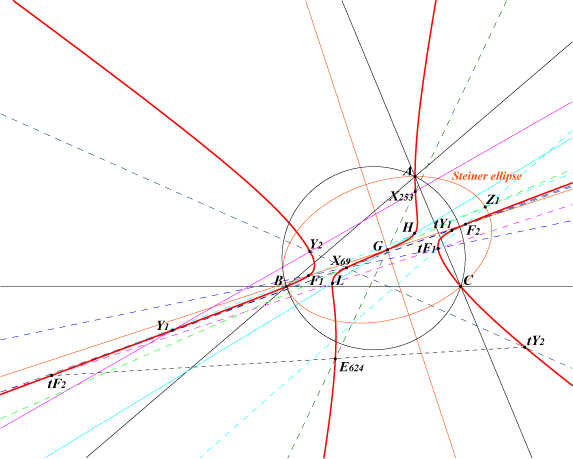More informations in Wilson's page. See the related cubic K709.Generalization of the cyclocevian conjugation Let (Γ) be the circum-conic with perspector M. Let P be a finite point with cevian triangle PaPbPc. There is one and only conic (C) passing through Pa, Pb, Pc and the infinite points of (Γ). (C) is a bicevian conic with perspectors P and another point Q we shall call the (Γ)-cevian conjugate of P. With M = p : q : r and P = x : y : z, the first barycentric coordinate of the isotomic conjugate of Q is given by : – p / (x(y + z)) + q / (y(z + x)) + r / (z(x + y)) Note that (Γ) and (C) must meet again at two (real or not) finite points which lie on the trilinear polar of the barycentric product P x Q. Obviously, with M = X(6), (Γ) is the circumcircle of ABC and Q is the cyclocevian conjugate of P. Q = f(M, P) = ta(M ÷ ctP) where ÷ denotes a barycentric quotient and g, t, c, a denote an isogonal conjugate, an isotomic conjugate, a complement, an anticomplement as usual. In other words, if mX denotes the M-isoconjugate of X, we obtain Q = tamctP. In particular, with M = X(6), we find Q = tagctP, as found a long time ago by Darij Grinberg (Hyacinthos #6423, January 24, 2003). See also Wilson's page mentioned above. Now, let (K) be the isotomic pivotal cubic pK(X2, S). For any point X on (K), the point Y = f(cS, X) also lies on (K) and the line XY passes through atS which can be seen as another pivot on the curve. atS is the P-Ceva conjugate of X(2). With S = X(69), (K) is the Lucas cubic K007, cS = X(6) and atS = X(20) which is property 5 above.Lucas triangle and Lucas isogonal conjugation K007 meets the circumcircle at A, B, C and three other points L1, L2, L3 on the rectangular hyperbola (H) passing through X(2), X(20), X(54), X(69), X(110), X(2574), X(2575), X(2979). These are the vertices of the Lucas triangle LT whose orthocenter is X(2979). LT is always an acutangle triangle. Its centroid is X = a^2 (a^6 b^2-3 a^4 b^4+3 a^2 b^6-b^8+a^6 c^2-11 a^4 b^2 c^2+9 a^2 b^4 c^2+b^6 c^2-3 a^4 c^4+9 a^2 b^2 c^4+3 a^2 c^6+b^2 c^6-c^8) : : , on the lines {3, 54}, {51, 631}, SEARCH = 7.07052700014795. X is obviously the image of X(2979) under the homothety h(O, 1/3). Note that (E) = {3, 54} is the Euler line of LT. X is now X(54041) in ETC. The Lemoine point of LT is X(54043).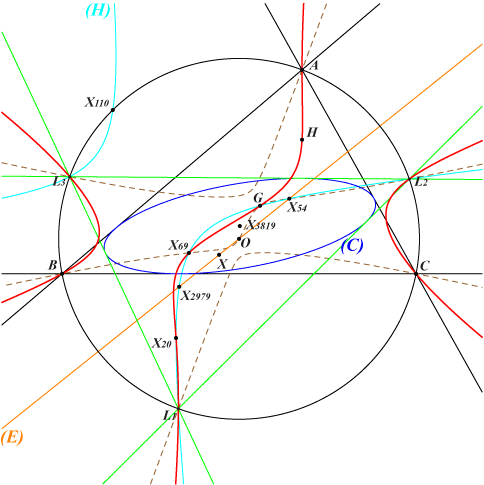The conic (C) is inscribed in both triangles ABC and LT. Its center is X(3819) and its perspector is X(34384). *** Under isogonal conjugation with respect to LT, every line passing through O is transformed into a rectangular circum-hyperbola of LT passing through X(2979). The hyperbola (H) above is the transform of the Euler line of ABC. The hyperbolas through {X22, X30, X476, X523, X2979} and {X329, X513, X517, X901, X2979} are the transforms of the lines through X(74), X(100) respectively. All these hyperbolas have their center on the nine points circle of LT, with radius R/2 and with center the midpoint of {X3,X2979}, on the lines {X3,X54}, {X51,X140}, {X143,X631}. This point is now X(54042) in ETC. *** pairs {P,Q} of isogonal conjugates with respect to LT : {2,20}, {3,2979}, {4,11206}, {8,9778}, {22,11459}, {54,52397}, {69,376}, {95,36987}, {329,5731}, {3534,38397}, {5890,20477}, {15531,21312}, {39158,39159}, {39160,39161}. Note that the last four points are the foci of the Steiner ellipse which lie on K007. For every point on the line at infinity or on the circumcircle, the isogonal conjugate with respect to LT coincide with the Thomson isogonal conjugate. *** Every pK(Ω, P) that passes through L1, L2, L3 must have • its pole Ω on psK(X54034, X95, X2) passing through X(2), X(6), X(97), X(4) × X(11206), X(22) × X(54), • its pivot P on psK(X95, X34384, X4) = spK(X51, X3819) passing through X(4), X(54), X(69), X(95) , X(2979), X(18018), X(275) × X(1370). Examples : K646 = pK(X97, X95), pK(X6, X2979). See also the related cubic K1326.